# Forecasting with ARIMA Modeling in R - Case Study

In this lesson, we will take a new dataset (stock prices) and use all that we have learned to create a forecast using the ARIMA Models.

We will take the closing prices of Facebook stock for this example.

### Step 1: Load the Data

We will load Facebook daily closing prices for the past 3 years from the WIKI/PRICES dataset in Quandl.

The following code loads the data from Quandl and converts it into a time series object called fb_ts.

Quandl.api\_key("YOUR API KEY")
fb_data <- Quandl.datatable("WIKI/PRICES" ,
qopts.columns=c("date", "close"),
ticker=c("FB"),
date.gte=c("2014-01-01"),
date.lte=c("2016-12-31"))
ZOO <- zoo(fb_data$close, order.by=as.Date(as.character(fb_data$date), format='%Y-%m-%d'))
fb_ts <- ts(ZOO)


### Step 2: Plot the Time Series and Test for Stationarity

We can now plot the time series and look for common patterns.

> plot.ts(fb_ts, main="Facebook Stock Prices", col=4)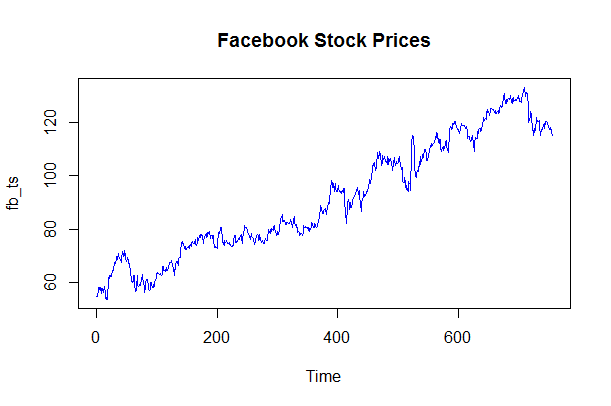The above chart exhibits a clear upward trend in the stock prices. This means that we will be differencing to make the series stationary.

Sometimes a visual inspection may not clearly identify such pattern. In such cases, we can use the Augmented Dickey-Fuller unit root test to check whether the series is stationary or not. The p-value resulting from the ADF test must be less than 0.05 or 5% for a time series to be stationary. If the p-value is greater than 0.05 or 5%, you conclude that the time series has a unit root which means that it is a non-stationary process.

In R, we can do this using adf.test() function available in the tseries package. The following code loads the required package and performs the test.

> install.packages("tseries")
> library(tseries)
Augmented Dickey-Fuller Test
data:  fb_ts
Dickey-Fuller = -3.1368, Lag order = 9, p-value = 0.09883
alternative hypothesis: stationary
>


The test results confirm our observation that series is non-stationary (p-value >0.05) and will need differencing to make it stationary.

### Step 3: Identify the Model

The next step is to identify the model, i.e., the appropriate order of Autoregressive (AR) and Moving Average (MA) processes p, and q. We will do so using the Autocorrelation function (ACF) and Partial Autocorrelation function (PACF).

Let's create the ACF and PACF plots.

> acf(fb_ts)
> pacf(fb_ts)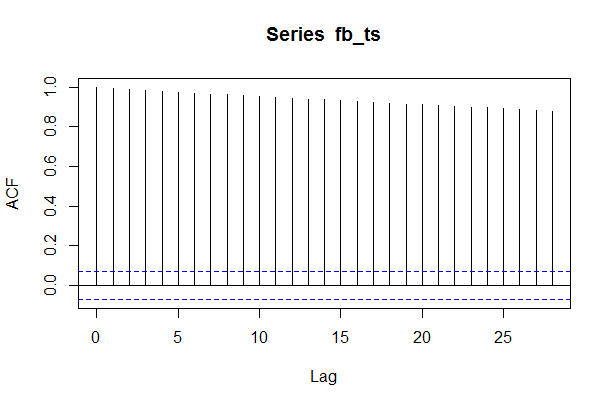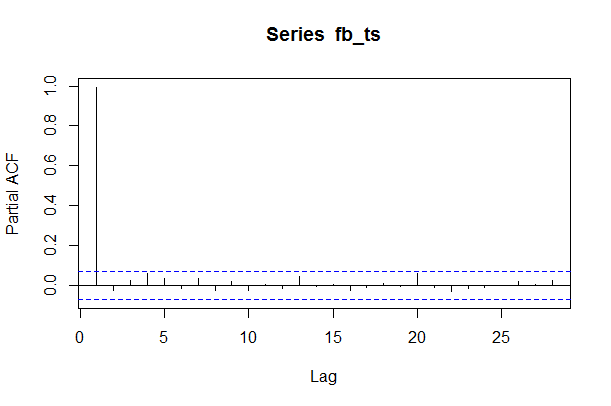Recall our analysis of these two functions. The ACF plot shows slow decay of lag to 0 indicating an AR model. The PACF plot suggests AR model of the order 1 AR(1) as PACF number is close to 0 after lag 1.

Identifying the best fit model is a complex process and we may want to test multiple models to check what best fits our data. Both experience and knowledge of advanced topics can be helpful. However, based on our limited analysis, let's say we will go with p=1, d=1, and q=0.

Our suggested model is then ARIMA(1,1,0).

### Step 4: Estimate the Model

We can now estimate the model for our data as shown below:

fb\_fit <- arima(fb\_ts, order = c(1, 1, 0))

> fb_fit
Call:
arima(x = fb_ts, order = c(1, 1, 0))
Coefficients:
ar1
0.0306
s.e.  0.0364
sigma^2 estimated as 2.642:  log likelihood = -1438.1,  aic = 2880.2
>


### Step 5: Create Forecast

The next step is to create a forecast using the fitted model. We are predicting the stock prices for the next 20 days.

> fb_forecast <- predict(fb_fit , n.ahead = 20)

> fb_forecast
$pred Time Series: Start = 757 End = 776 Frequency = 1  115.0102 115.0090 115.0089 115.0089 115.0089 115.0089 115.0089 115.0089 115.0089  115.0089 115.0089 115.0089 115.0089 115.0089 115.0089 115.0089 115.0089 115.0089  115.0089 115.0089$se
Time Series:
Start = 757
End = 776
Frequency = 1
 1.625520 2.334309 2.874161 3.327575 3.726221 4.086157 4.416859 4.724469 5.013239
 5.286259 5.545854 5.793829 6.031618 6.260382 6.481076 6.694498 6.901324 7.102128
 7.297410 7.487600
>


Notice that all predicted values for the next 20 periods look the same. This is because the forecast will be flat with no drift. There is a possibility that our model has a drift also along with a trend.

For simplicity sake, let's also extract the two series in their own respective variables.

> fb_forecast_values <- fb_forecast$pred > fb_forecast_se <- fb_forecast$se


### Step 6: Plot the Forecast

We can now plot the original series and the forecast as shown below:

> plot.ts(fb_ts, xlim = c(0, 900), ylim = c(50,160))
> points(fb_forecast_values , type = "l", col = 2)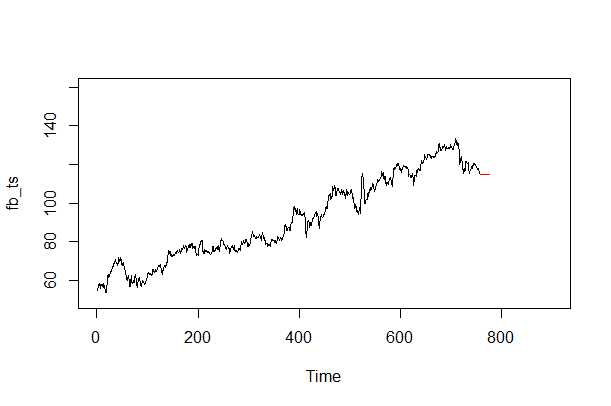We can also plot the fitted data for the current time period on the chart to show how well the model fits the data.

> fb_fitted_data <- fb_ts - residuals(fb_fit)
> points(fb_fitted_data, type = "l", col = 4, lty = 2)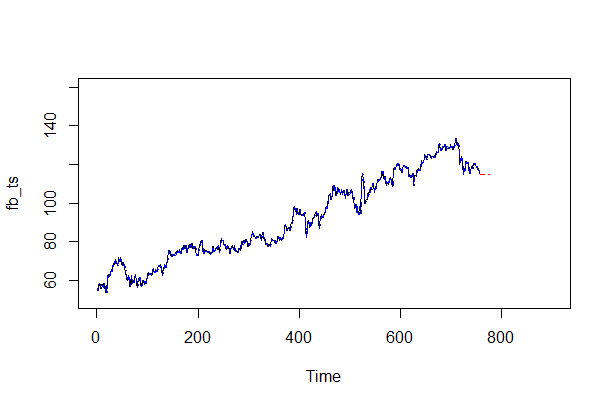Get our Data Science for Finance Bundle for just $29$51.